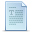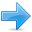# Polymorphism

Before getting any deeper into this chapter, you should have a proper understanding of pointers and class inheritance. If you are not really sure of the meaning of any of the following expressions, you should review the indicated sections:

Statement:Explained in:
`int A::b(int c) { }`Classes
`a->b`Data structures
`class A: public B {};`Friendship and inheritance

### Pointers to base class

One of the key features of class inheritance is that a pointer to a derived class is type-compatible with a pointer to its base class. Polymorphism is the art of taking advantage of this simple but powerful and versatile feature.

The example about the rectangle and triangle classes can be rewritten using pointers taking this feature into account:

 ``````// pointers to base class #include using namespace std; class Polygon { protected: int width, height; public: void set_values (int a, int b) { width=a; height=b; } }; class Rectangle: public Polygon { public: int area() { return width*height; } }; class Triangle: public Polygon { public: int area() { return width*height/2; } }; int main () { Rectangle rect; Triangle trgl; Polygon * ppoly1 = ▭ Polygon * ppoly2 = &trgl; ppoly1->set_values (4,5); ppoly2->set_values (4,5); cout << rect.area() << '\n'; cout << trgl.area() << '\n'; return 0; }`````` ```20 10```

Function `main` declares two pointers to `Polygon` (named `ppoly1` and `ppoly2`). These are assigned the addresses of `rect` and `trgl`, respectively, which are objects of type `Rectangle` and `Triangle`. Such assignments are valid, since both `Rectangle` and `Triangle` are classes derived from `Polygon`.

Dereferencing `ppoly1` and `ppoly2` (with `ppoly1->` and `ppoly2->`) is valid and allows us to access the members of their pointed objects. For example, the following two statements would be equivalent in the previous example:

 ``12`` ``````ppoly1->set_values (4,5); rect.set_values (4,5);``````

But because the type of both `ppoly1` and `ppoly2` is pointer to `Polygon` (and not pointer to `Rectangle` nor pointer to `Triangle`), only the members inherited from `Polygon` can be accessed, and not those of the derived classes `Rectangle` and `Triangle`. That is why the program above accesses the `area` members of both objects using `rect` and `trgl` directly, instead of the pointers; the pointers to the base class cannot access the `area` members.

Member `area` could have been accessed with the pointers to `Polygon` if `area` were a member of `Polygon` instead of a member of its derived classes, but the problem is that `Rectangle` and `Triangle` implement different versions of `area`, therefore there is not a single common version that could be implemented in the base class.

### Virtual members

A virtual member is a member function that can be redefined in a derived class, while preserving its calling properties through references. The syntax for a function to become virtual is to precede its declaration with the `virtual` keyword:

 ``````// virtual members #include using namespace std; class Polygon { protected: int width, height; public: void set_values (int a, int b) { width=a; height=b; } virtual int area () { return 0; } }; class Rectangle: public Polygon { public: int area () { return width * height; } }; class Triangle: public Polygon { public: int area () { return (width * height / 2); } }; int main () { Rectangle rect; Triangle trgl; Polygon poly; Polygon * ppoly1 = ▭ Polygon * ppoly2 = &trgl; Polygon * ppoly3 = &poly; ppoly1->set_values (4,5); ppoly2->set_values (4,5); ppoly3->set_values (4,5); cout << ppoly1->area() << '\n'; cout << ppoly2->area() << '\n'; cout << ppoly3->area() << '\n'; return 0; }`````` ```20 10 0```

In this example, all three classes (`Polygon`, `Rectangle` and `Triangle`) have the same members: `width`, `height`, and functions `set_values` and `area`.

The member function `area` has been declared as `virtual` in the base class because it is later redefined in each of the derived classes. Non-virtual members can also be redefined in derived classes, but non-virtual members of derived classes cannot be accessed through a reference of the base class: i.e., if `virtual` is removed from the declaration of `area` in the example above, all three calls to `area` would return zero, because in all cases, the version of the base class would have been called instead.

Therefore, essentially, what the `virtual` keyword does is to allow a member of a derived class with the same name as one in the base class to be appropriately called from a pointer, and more precisely when the type of the pointer is a pointer to the base class that is pointing to an object of the derived class, as in the above example.

A class that declares or inherits a virtual function is called a polymorphic class.

Note that despite of the virtuality of one of its members, `Polygon` was a regular class, of which even an object was instantiated (`poly`), with its own definition of member `area` that always returns 0.

### Abstract base classes

Abstract base classes are something very similar to the `Polygon` class in the previous example. They are classes that can only be used as base classes, and thus are allowed to have virtual member functions without definition (known as pure virtual functions). The syntax is to replace their definition by `=0` (an equal sign and a zero):

An abstract base `Polygon` class could look like this:

 ``123456789`` ``````// abstract class CPolygon class Polygon { protected: int width, height; public: void set_values (int a, int b) { width=a; height=b; } virtual int area () =0; };``````

Notice that `area` has no definition; this has been replaced by `=0`, which makes it a pure virtual function. Classes that contain at least one pure virtual function are known as abstract base classes.

Abstract base classes cannot be used to instantiate objects. Therefore, this last abstract base class version of `Polygon` could not be used to declare objects like:

 ``1`` ``Polygon mypolygon; // not working if Polygon is abstract base class ``

But an abstract base class is not totally useless. It can be used to create pointers to it, and take advantage of all its polymorphic abilities. For example, the following pointer declarations would be valid:

 ``12`` ``````Polygon * ppoly1; Polygon * ppoly2;``````

And can actually be dereferenced when pointing to objects of derived (non-abstract) classes. Here is the entire example:

 ``````// abstract base class #include using namespace std; class Polygon { protected: int width, height; public: void set_values (int a, int b) { width=a; height=b; } virtual int area (void) =0; }; class Rectangle: public Polygon { public: int area (void) { return (width * height); } }; class Triangle: public Polygon { public: int area (void) { return (width * height / 2); } }; int main () { Rectangle rect; Triangle trgl; Polygon * ppoly1 = ▭ Polygon * ppoly2 = &trgl; ppoly1->set_values (4,5); ppoly2->set_values (4,5); cout << ppoly1->area() << '\n'; cout << ppoly2->area() << '\n'; return 0; }`````` ```20 10```

In this example, objects of different but related types are referred to using a unique type of pointer (`Polygon*`) and the proper member function is called every time, just because they are virtual. This can be really useful in some circumstances. For example, it is even possible for a member of the abstract base class `Polygon` to use the special pointer `this` to access the proper virtual members, even though `Polygon` itself has no implementation for this function:

 ``````// pure virtual members can be called // from the abstract base class #include using namespace std; class Polygon { protected: int width, height; public: void set_values (int a, int b) { width=a; height=b; } virtual int area() =0; void printarea() { cout << this->area() << '\n'; } }; class Rectangle: public Polygon { public: int area (void) { return (width * height); } }; class Triangle: public Polygon { public: int area (void) { return (width * height / 2); } }; int main () { Rectangle rect; Triangle trgl; Polygon * ppoly1 = ▭ Polygon * ppoly2 = &trgl; ppoly1->set_values (4,5); ppoly2->set_values (4,5); ppoly1->printarea(); ppoly2->printarea(); return 0; }`````` ```20 10```

Virtual members and abstract classes grant C++ polymorphic characteristics, most useful for object-oriented projects. Of course, the examples above are very simple use cases, but these features can be applied to arrays of objects or dynamically allocated objects.

Here is an example that combines some of the features in the latest chapters, such as dynamic memory, constructor initializers and polymorphism:

 ``````// dynamic allocation and polymorphism #include using namespace std; class Polygon { protected: int width, height; public: Polygon (int a, int b) : width(a), height(b) {} virtual int area (void) =0; void printarea() { cout << this->area() << '\n'; } }; class Rectangle: public Polygon { public: Rectangle(int a,int b) : Polygon(a,b) {} int area() { return width*height; } }; class Triangle: public Polygon { public: Triangle(int a,int b) : Polygon(a,b) {} int area() { return width*height/2; } }; int main () { Polygon * ppoly1 = new Rectangle (4,5); Polygon * ppoly2 = new Triangle (4,5); ppoly1->printarea(); ppoly2->printarea(); delete ppoly1; delete ppoly2; return 0; }`````` ```20 10```

Notice that the `ppoly` pointers:

 ``12`` ``````Polygon * ppoly1 = new Rectangle (4,5); Polygon * ppoly2 = new Triangle (4,5);``````

are declared being of type "pointer to `Polygon`", but the objects allocated have been declared having the derived class type directly (`Rectangle` and `Triangle`).Previous:Friendship and inheritanceIndexNext:Type conversions